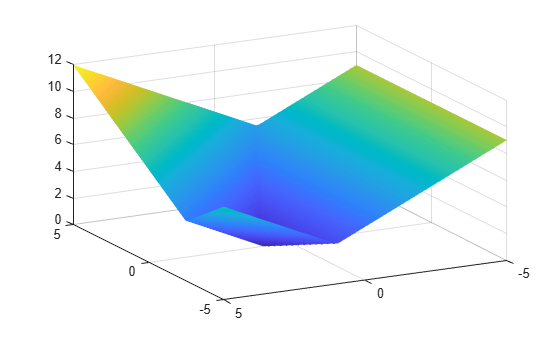Compare fminimax and fminunc

A minimax problem minimizes the maximum of a set of objective functions. Why not minimize this maximum function, which is a scalar function? The answer is that the maximum is not smooth, and Optimization Toolbox™ solvers such as fminunc require smoothness.

For example, define fun(x) as three linear objective functions in two variables, and fun2 as the maximum of these three objectives.

a = [1;1];
b = [-1;1];
c = [0;-1];
a0 = 2;
b0 = -3;
c0 = 4;
fun = @(x)[x*a+a0,x*b+b0,x*c+c0];
fun2 = @(x)max(fun(x),[],2);

Plot the maximum of the three objectives.

[X,Y] = meshgrid(linspace(-5,5));
Z = fun2([X(:),Y(:)]);
Z = reshape(Z,size(X));
surf(X,Y,Z,'LineStyle','none')
view(-118,28)fminimax finds the minimax point easily.

x0 = [0,0];
[xm,fvalm,maxfval] = fminimax(fun,x0)
Local minimum possible. Constraints satisfied.

fminimax stopped because the size of the current search direction is less than
twice the value of the step size tolerance and constraints are
satisfied to within the value of the constraint tolerance.
xm = 1×2

-2.5000    2.2500

fvalm = 1×3

1.7500    1.7500    1.7500

maxfval = 1.7500

However, fminunc stops at a point that is far from the minimax point.

[xu,fvalu] = fminunc(fun2,x0)
Local minimum possible.

fminunc stopped because it cannot decrease the objective function
along the current search direction.
xu = 1×2

0    1.0000

fvalu = 3.0000

fminimax finds a better (smaller) solution.

fprintf("fminimax finds a point with objective %g,\nwhile fminunc finds a point with objective %g.",maxfval,fvalu)
fminimax finds a point with objective 1.75,
while fminunc finds a point with objective 3.